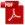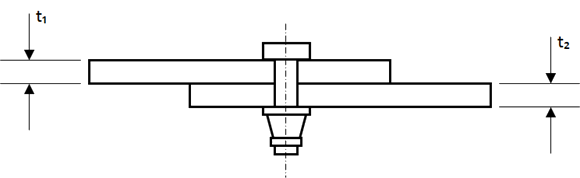### 7.4. Fastener Shear Stiffness

Reference: Abbott, Richard. Analysis and Design of Composite and Metallic Flight Vehicle Structures 3 Edition, 2019

Fastener shear stiffness or fastener joint stiffness’ are often used in bolt group models and finite element models. It is important to note that at best, fastener stiffness values are approximations. The stiffness of a joint and each fastener within the joint depends on many factors including – the condition of the joint surfaces, the clamp-up force in the joint, the clearance of the fasteners in the fastener holes, the material of the fastener, the material of the sheet, the thickness of the sheets, the size of the fastener, the fastener head size, the nut type, whether washers are used, etc.

There are a range of methods of varying complexity to calculate fastener stiffness’ for use in joint analyses and they are all approximations.

It is recommended that a relatively simple approach is used.

(NACA-TN-1051, 1946) provides a rational method and experimental derivation for the critical parameters.

The flexibility of a single fastener is given by the following expression:

Where:

Average Joint Plate Thickness for a single shear joint:Figure 7.3.3‑1: Average Thickness for a Single Shear Joint

Average Joint Plate Thickness for a Double Shear Joint:Figure 7.3.3‑2: Average Thickness for a Double Shear Joint

### 7.4. Fastener Shear Stiffness

Reference: Abbott, Richard. Analysis and Design of Composite and Metallic Flight Vehicle Structures 3 Edition, 2019

Fastener shear stiffness or fastener joint stiffness’ are often used in bolt group models and finite element models. It is important to note that at best, fastener stiffness values are approximations. The stiffness of a joint and each fastener within the joint depends on many factors including – the condition of the joint surfaces, the clamp-up force in the joint, the clearance of the fasteners in the fastener holes, the material of the fastener, the material of the sheet, the thickness of the sheets, the size of the fastener, the fastener head size, the nut type, whether washers are used, etc.

There are a range of methods of varying complexity to calculate fastener stiffness’ for use in joint analyses and they are all approximations.

It is recommended that a relatively simple approach is used.

(NACA-TN-1051, 1946) provides a rational method and experimental derivation for the critical parameters.

The flexibility of a single fastener is given by the following expression:

Where:

Average Joint Plate Thickness for a single shear joint:Figure 7.3.3‑1: Average Thickness for a Single Shear Joint

Average Joint Plate Thickness for a Double Shear Joint:Figure 7.3.3‑2: Average Thickness for a Double Shear Joint